Chapter 4 Part 1 - AD,AS and Related Concepts

Economics Class 12
Macroeconomics

## What is APS?

APC means Average Propensity to Save

It is Ratio of saving to corresponding level of income

Average Propensity to Save = Savings/ Income

APS = S/Y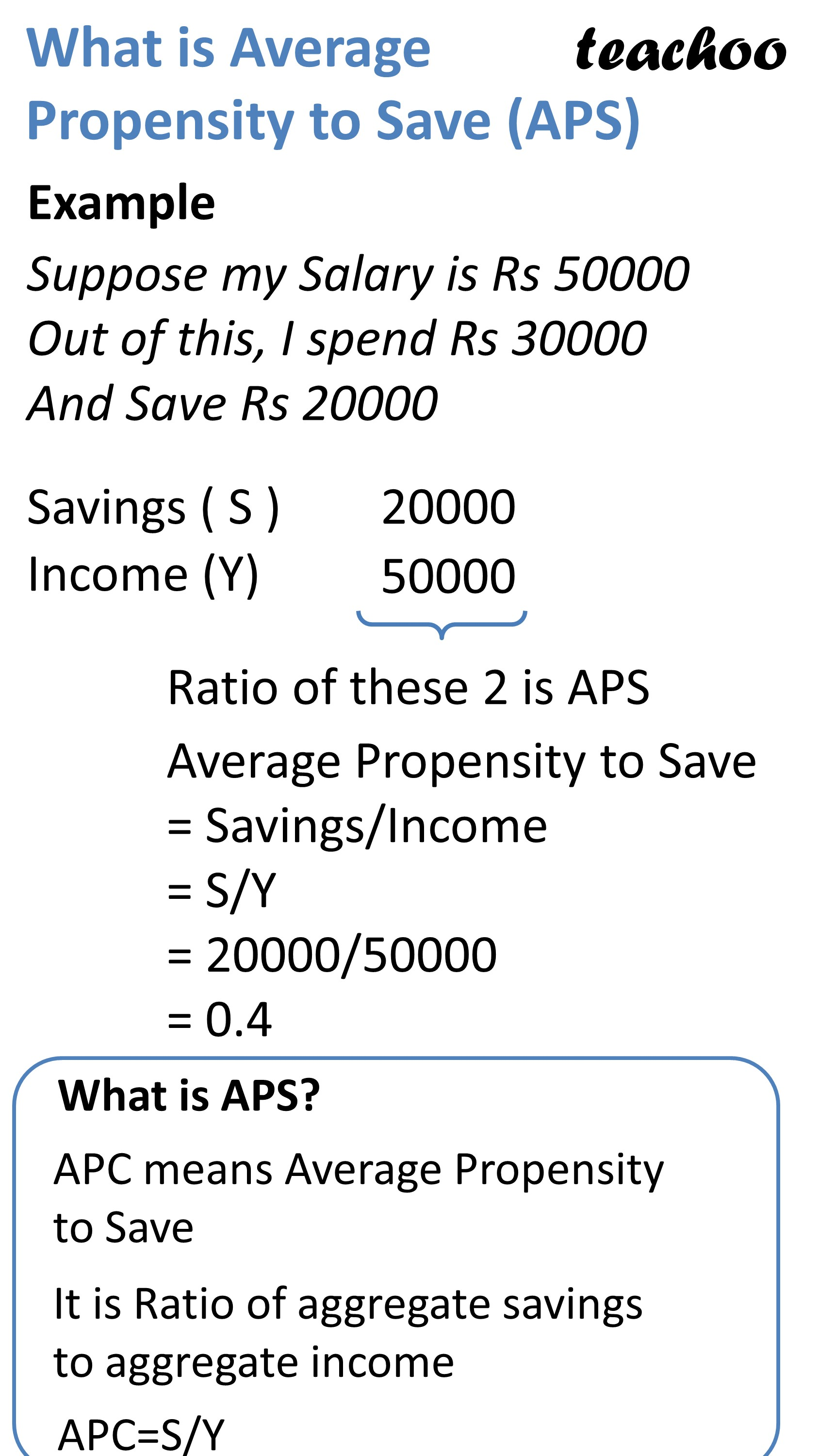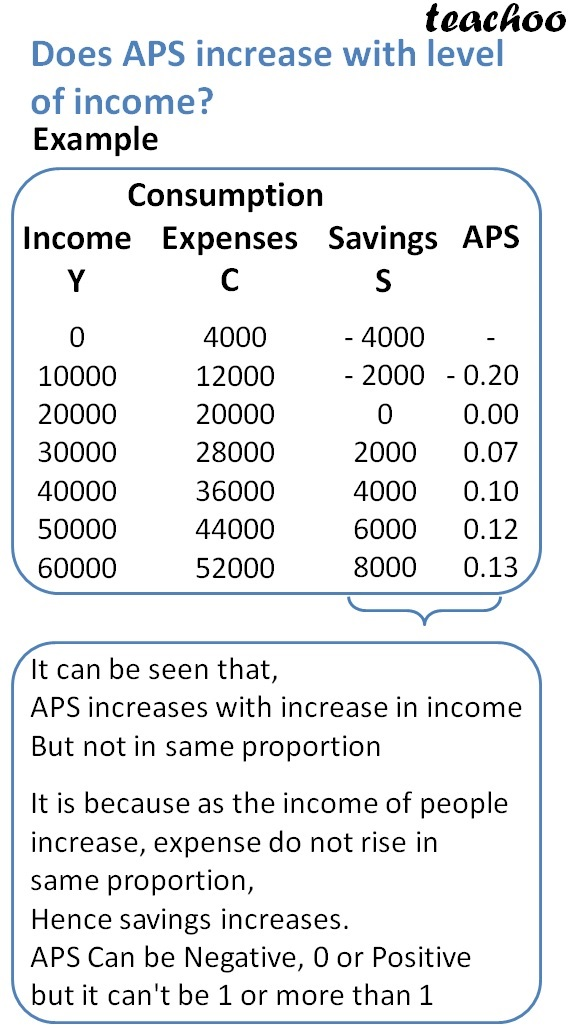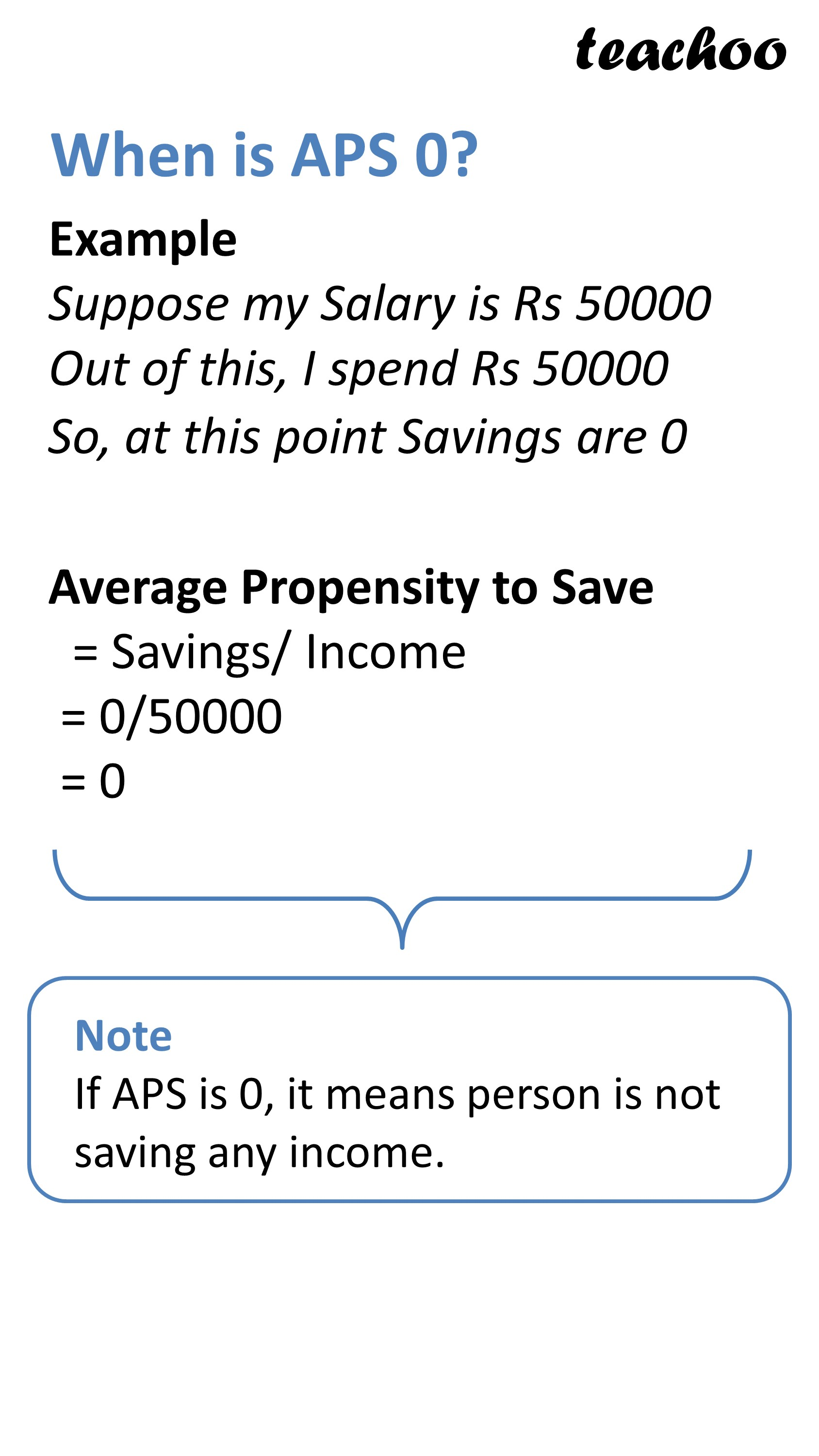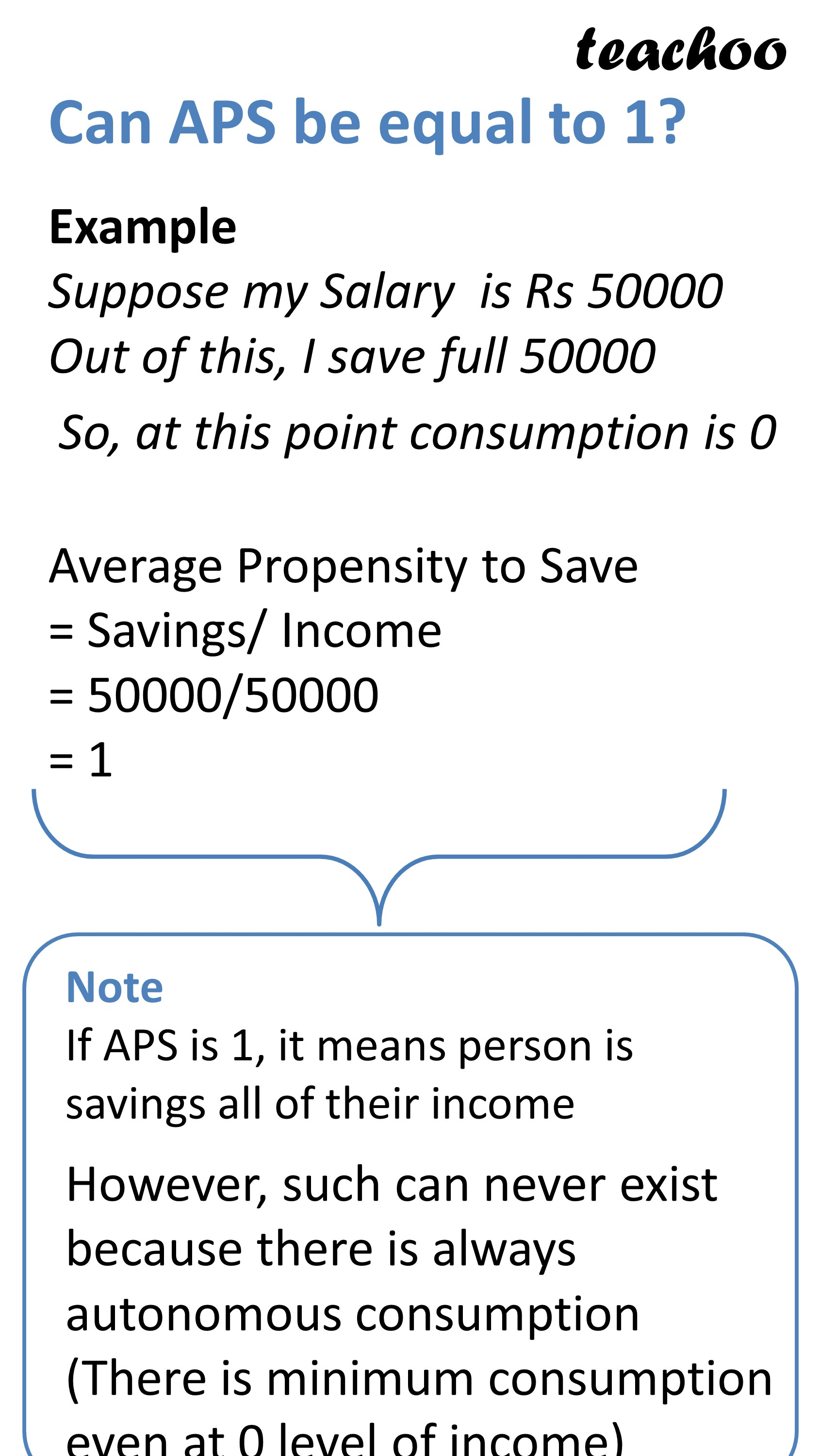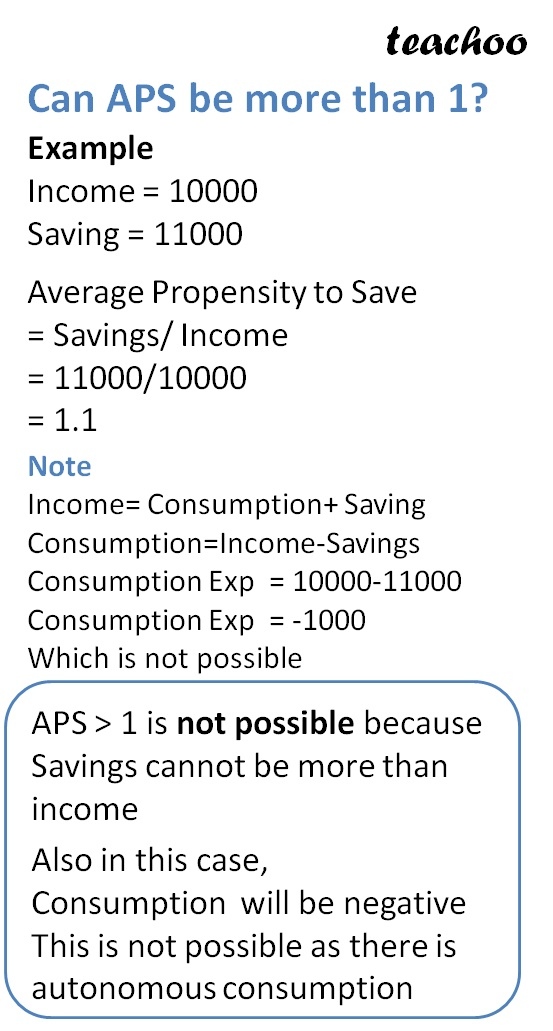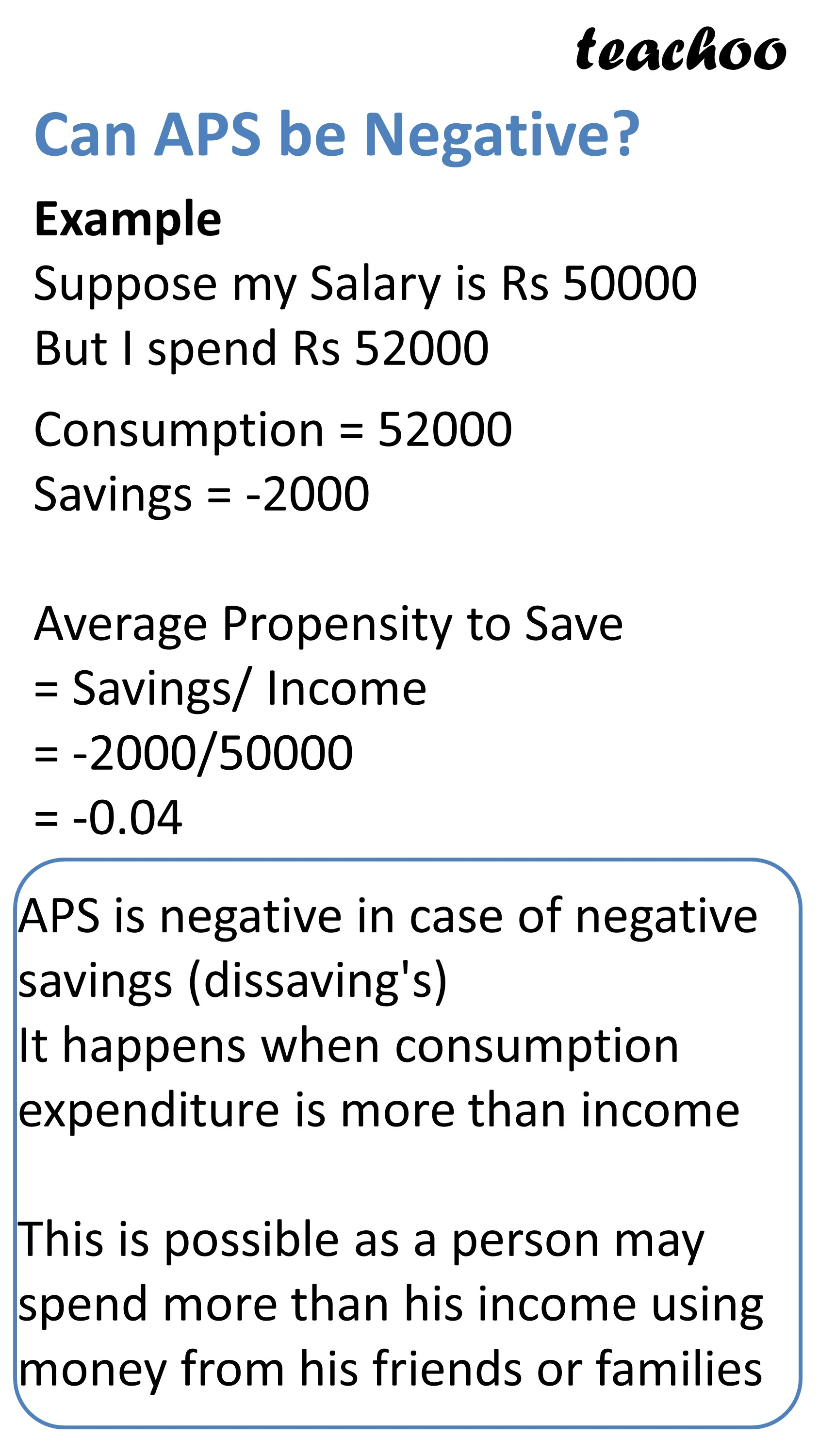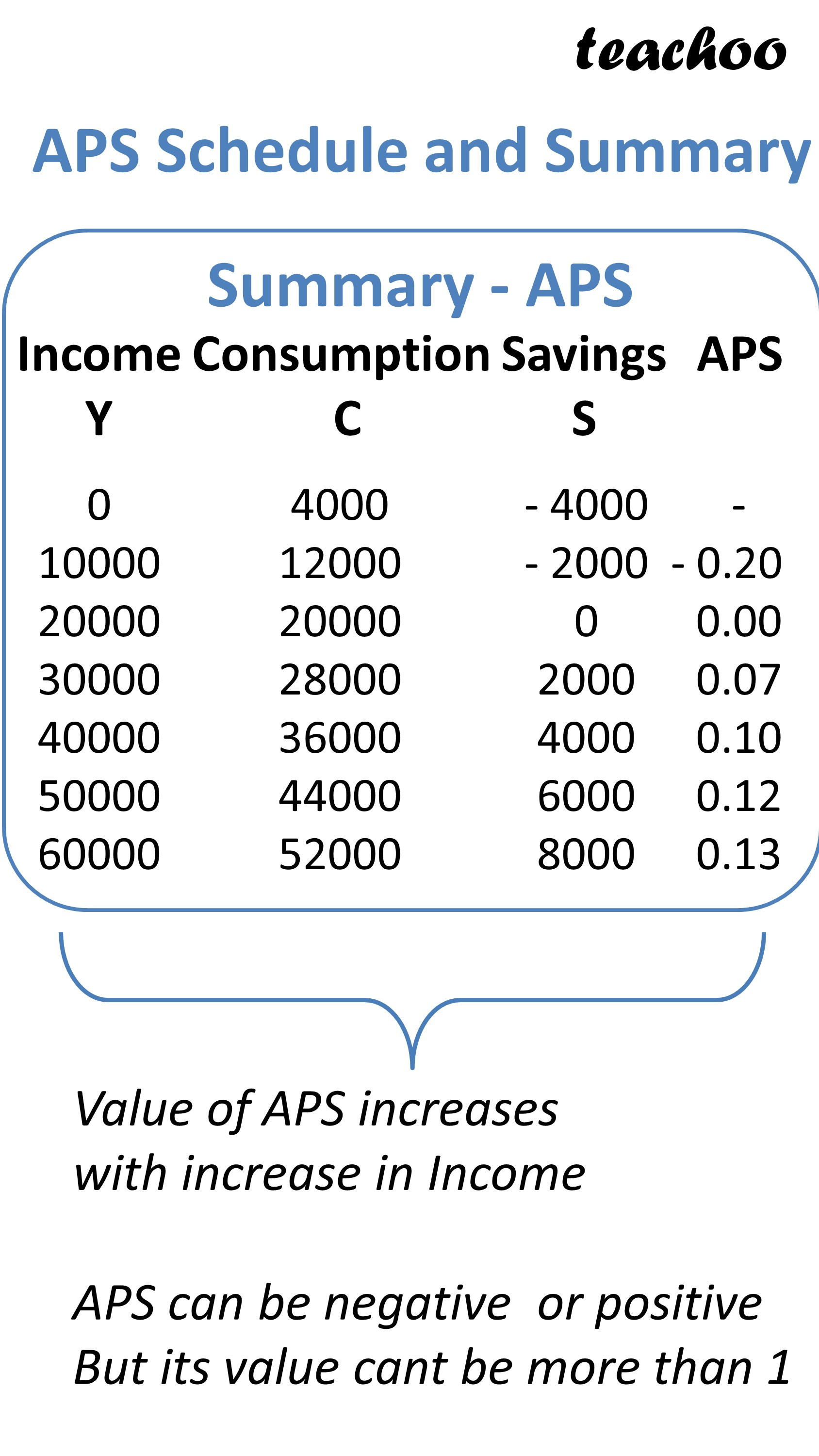Income Consumption Expenses Savings APS Y C S 0 4000 -4000 - 10000 12000 -2000 -0.20 20000 20000 0 0.00 30000 28000 2000 0.07 40000 36000 4000 0.10 50000 44000 6000 0.12 60000 52000 8000 0.13

APS Increase with level of Income

It is because as the income of people increase, income does not rise with same proportion

APS Can be Negative, 0 or Positive but it can't be 1 or more than 1

When is APS Negative?

It is negative in case of negative savings (dissavings)

It happens when consumption expenditure is more than income

When is APS = 0?

It happens when consumption expense is equal to income and there are zero savings

## Can APS Be 1 or more than 1?

APS Can be 1 only IF

Savings = Income

and there is no consumption expenditure

This is not possible because there is autonomous consumption expenditure

Example

Suppose Income = 10000

Consumption Exp = 0

Saving = 10000 - 0 = 10000

In this case, APS = 10000/10000 = 1

This is not possible because consumption exp cannot be 0

Similarly APS > 1 is not possible because Savings cannot be more than income

Example

Suppose Income = 10000

Consumption Exp = -1000

Saving = 10000 - 0 = 11000

This is not possible as consumption exp cannot be negative

## How to draw Graph of APS

On X Axis, We represent Income

On Y Axis, We Represent Savings

It can be seen that

Savings Curve is Positively Sloping

However it stats at Point S which is below the origin

At any point of Consumption curve, we can calculate APS

Suppose we are calculating at Point A

APC = Savings/Income

APS = OR/OY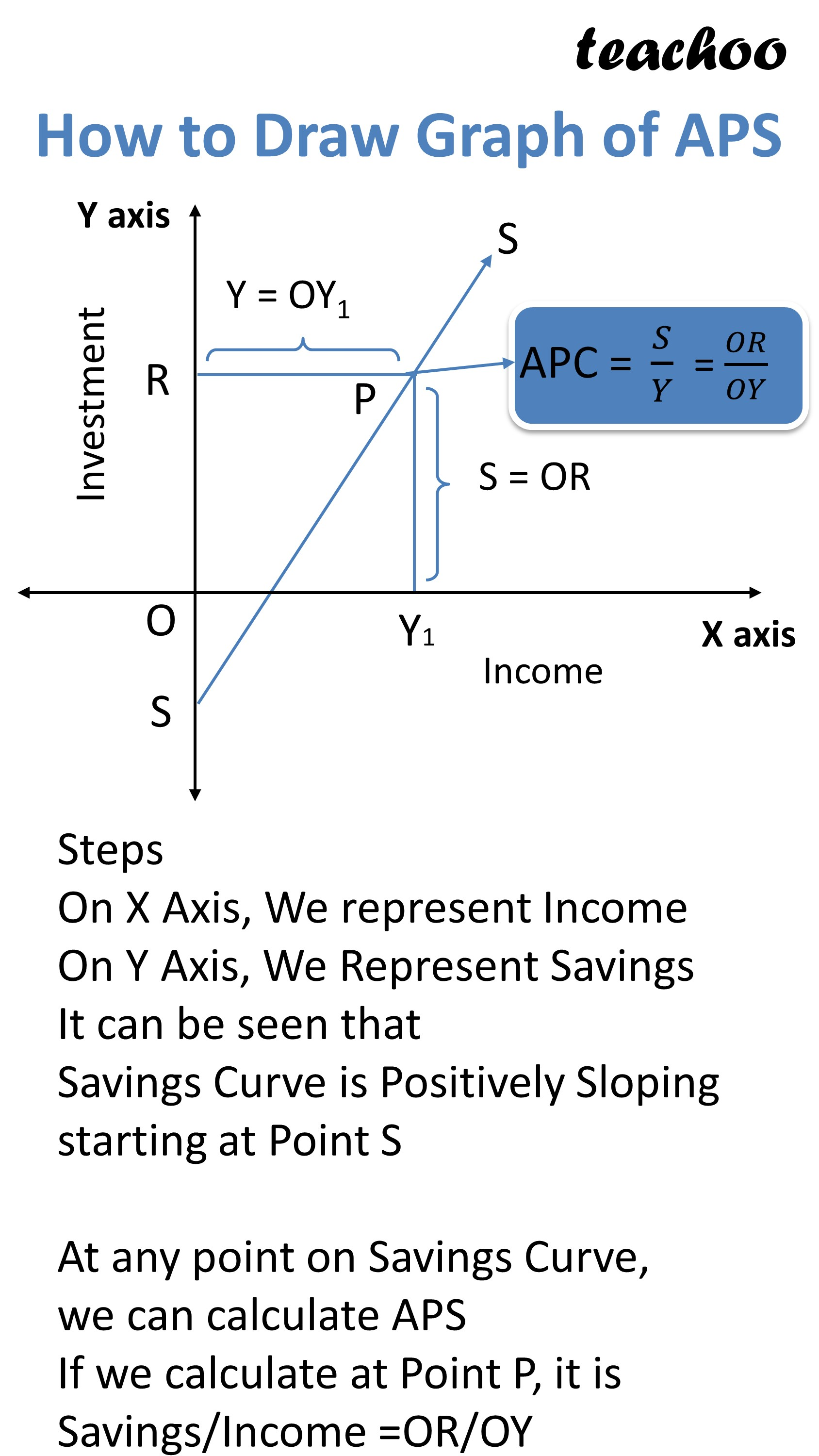### NCERT Questions

No questions in this part

### Other Books

#### Question 1

What is APS?

State some important points.

Get live Maths 1-on-1 Classs - Class 6 to 12

### Transcript

What is Average Propensity to Save (APS) Example Suppose my Salary is Rs 50000 Out of this, I spend Rs 30000 And Save Rs 20000 Savings ( S ) Income (Y) 20000 50000 Ratio of these 2 is APS Average Propensity to Save = Savings/Income = S/Y = 20000/50000 = 0.4 What is APS? APC means Average Propensity to Save It is Ratio of aggregate savings to aggregate income APC=S/Y Does APS increase with level of income? Example Consumption Expenses C Income Y Savings S APS 0 10000 20000 30000 40000 50000 60000 4000 12000 20000 28000 36000 44000 52000 - 4000 - 2000 0 2000 4000 6000 8000 - - 0.20 0.00 0.07 0.10 0.12 0.13 It can be seen that, APS increases with increase in income But not in same proportion It is because as the income of people increase, income does not rise with same proportion APS Can be Negative, 0 or Positive but it can't be 1 or more than 1 When is APS 0? Example Suppose my Salary is Rs 50000 Out of this, I spend Rs 50000 So, at this point Savings are 0 Average Propensity to Save = Savings/ Income = 0/50000 = 0 Note If APS is 0, it means person is not saving any income. Can APS be equal to 1? Example Suppose my Salary is Rs 50000 Out of this, I save full 50000 So, at this point consumption is 0 Average Propensity to Save = Savings/ Income = 50000/50000 = 1 Note If APS is 1, it means person is savings all of their income However, such can never exist because there is always autonomous consumption (There is minimum consumption even at 0 level of income) Can APS be more than 1? Example Income = 10000 Saving = 11000 Average Propensity to Save = Savings/ Income = 11000/10000 = 1.1 Note Income= Consumption+ Saving Consumption=Income-Savings Consumption Exp = 10000-11000 Consumption Exp = -1000 Which is not possible APS > 1 is not possible because Savings cannot be more than income Also in this case, Consumption will be negative This is not possible as there is autonomous consumption Can APS be Negative? Example Suppose my Salary is Rs 50000 But I spend Rs 52000 Consumption = 52000 Savings = -2000 Average Propensity to Save = Savings/ Income = -2000/50000 = -0.04 APS is negative in case of negative savings (dissaving's) It happens when consumption expenditure is more than income This is possible as a person may spend more than his income using money from his friends or families APS Schedule and Summary Summary - APS Income Y Consumption C Savings S APS 0 10000 20000 30000 40000 50000 60000 4000 12000 20000 28000 36000 44000 52000 - 4000 - 2000 0 2000 4000 6000 8000 - - 0.20 0.00 0.07 0.10 0.12 0.13 Value of APS increases with increase in Income APS can be negative or positive But its value cant be more than 1 How to Draw Graph of APS Steps On X Axis, We represent Income On Y Axis, We Represent Savings It can be seen that Savings Curve is Positively Sloping starting at Point S At any point on Savings Curve, we can calculate APS If we calculate at Point P, it is Savings/Income =OR/OY